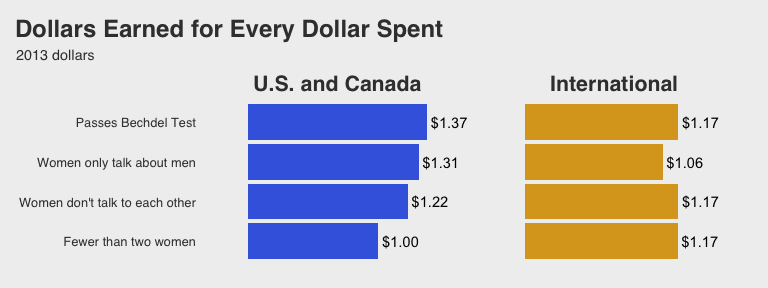# Bechdel analysis using the tidyverse

#### 2018-02-11

This vignette is based on tidyverse-ifying the R code here and reproducing some of the plots and analysis done in the 538 story entitled “The Dollar-And-Cents Case Against Hollywood’s Exclusion of Women” by Walt Hickey available here.

Load required packages to reproduce analysis. Also load the bechdel dataset for analysis.

library(fivethirtyeight)
# tidyverse includes ggplot2, tibble, tidyr, readr, purrr, dplyr:
library(tidyverse)
library(knitr)
library(magrittr)
library(broom)
library(stringr)
library(ggthemes)
library(scales)
# Turn off scientific notation
options(scipen = 99)

## Filter to only 1990 - 2013

Focus only on films from 1990 to 2013

bechdel90_13 <- bechdel %>% filter(between(year, 1990, 2013))

## Calculate variables

Create international gross only and return on investment (ROI) columns and add to bechdel_90_13 data frame

bechdel90_13 %<>%
mutate(int_only = intgross_2013 - domgross_2013,
roi_total = intgross_2013 / budget_2013,
roi_dom = domgross_2013 / budget_2013,
roi_int = int_only / budget_2013)

## Create generous variable

bechdel90_13 %<>%
mutate(generous = ifelse(test = clean_test %in% c("ok", "dubious"),
yes = TRUE,
no = FALSE))

## Determine median ROI and budget based on categories

ROI_by_binary <- bechdel90_13 %>%
group_by(binary) %>%
summarize(median_ROI = median(roi_total, na.rm = TRUE))
ROI_by_binary
binary median_ROI
FAIL 2.454209
PASS 2.696553
bechdel90_13 %>%
summarize(
Median Overall Return on Investment = median(roi_total, na.rm = TRUE))
Median Overall Return on Investment
2.569013
budget_by_binary <- bechdel90_13 %>%
group_by(binary) %>%
summarize(median_budget = median(budget_2013, na.rm = TRUE))
budget_by_binary
binary median_budget
FAIL 48385984
PASS 31070724
bechdel90_13 %>%
summarize(Median Overall Budget = median(budget_2013, na.rm = TRUE))
Median Overall Budget
37878971

## View Distributions

Look at the distributions of budget, international gross, ROI, and their logarithms

ggplot(data = bechdel90_13, mapping = aes(x = budget)) +
geom_histogram(color = "white", bins = 20) +
labs(title = "Histogram of budget")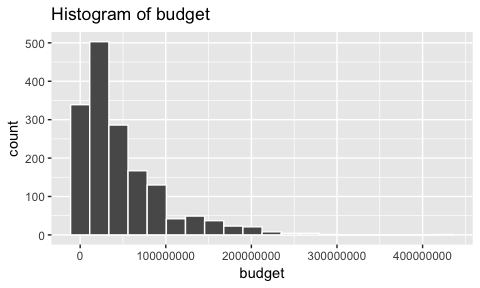ggplot(data = bechdel90_13, mapping = aes(x = log(budget))) +
geom_histogram(color = "white", bins = 20) +
labs(title = "Histogram of Logarithm of Budget")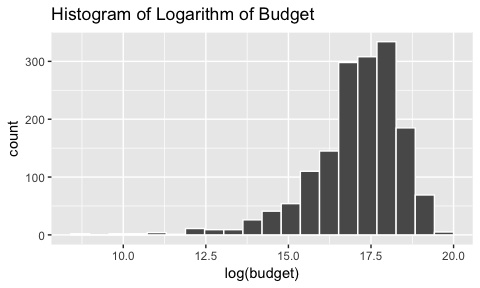ggplot(data = bechdel90_13, mapping = aes(x = intgross_2013)) +
geom_histogram(color = "white", bins = 20) +
labs(title = "Histogram of International Gross")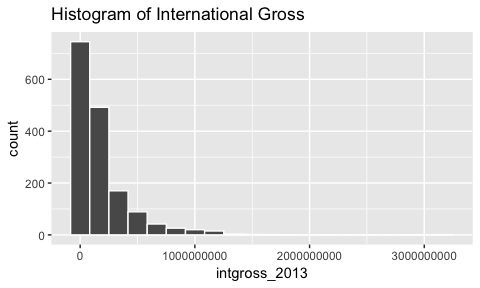ggplot(data = bechdel90_13, mapping = aes(x = log(intgross_2013))) +
geom_histogram(color = "white", bins = 20) +
labs(title = "Histogram of Logarithm of International Gross")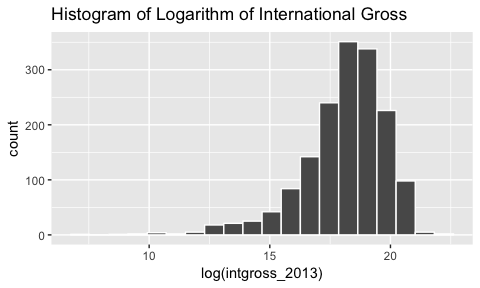ggplot(data = bechdel90_13, mapping = aes(x = roi_total)) +
geom_histogram(color = "white", bins = 20) +
labs(title = "Histogram of ROI")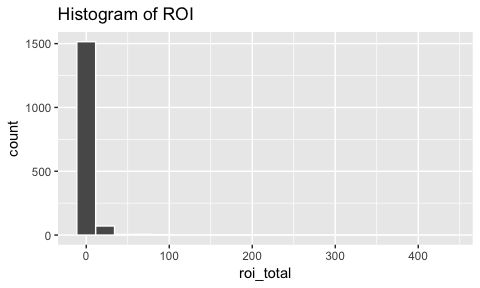The previous distributions were skewed, but ROI is so skewed that purposefully limiting the x-axis may reveal a bit more information about the distribution: (Suggested by Mustafa Ascha.)

ggplot(data = bechdel90_13, mapping = aes(x = roi_total)) +
geom_histogram(color = "white", bins = 20) +
labs(title = "Histogram of ROI") +
xlim(0, 25)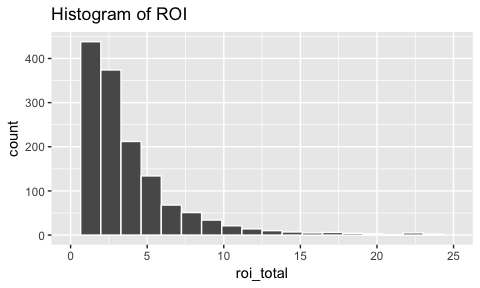ggplot(data = bechdel90_13, mapping = aes(x = log(roi_total))) +
geom_histogram(color = "white", bins = 20) +
labs(title = "Histogram of Logarithm of ROI")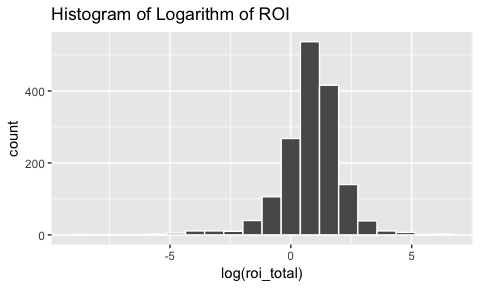## Linear Regression Models

### Movies with higher budgets make more international gross revenues using logarithms on both variables

ggplot(data = bechdel90_13,
mapping = aes(x = log(budget_2013), y = log(intgross_2013))) +
geom_point() +
geom_smooth(method = "lm", se = FALSE)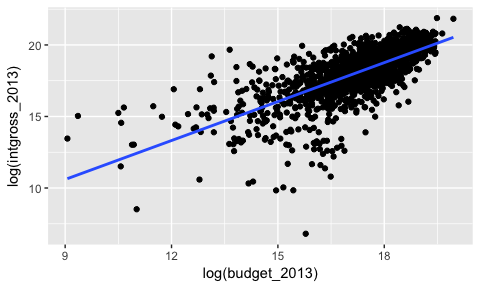gross_vs_budget <- lm(log(intgross_2013) ~ log(budget_2013),
data = bechdel90_13)
tidy(gross_vs_budget)
term estimate std.error statistic p.value
(Intercept) 2.4300342 0.3898688 6.232953 0
log(budget_2013) 0.9073902 0.0225334 40.268661 0

### Bechdel dummy is not a significant predictor of log(intgross_2013) assuming log(budget_2013) is in the model

Note that the regression lines nearly completely overlap.

ggplot(data = bechdel90_13,
mapping = aes(x = log(budget_2013), y = log(intgross_2013),
color = binary)) +
geom_point() +
geom_smooth(method = "lm", se = FALSE)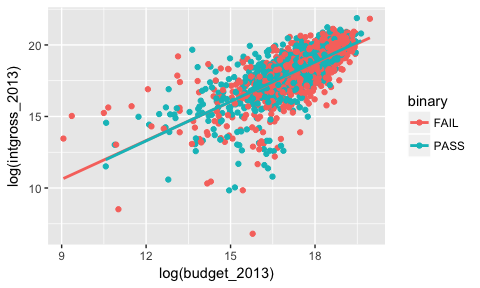gross_vs_budget_binary <- lm(log(intgross_2013) ~ log(budget_2013) + factor(binary),
data = bechdel90_13)
tidy(gross_vs_budget_binary)
term estimate std.error statistic p.value
(Intercept) 2.3585962 0.3988812 5.9130285 0.0000000
log(budget_2013) 0.9100772 0.0227566 39.9918064 0.0000000
factor(binary)PASS 0.0539207 0.0635194 0.8488861 0.3960713

Note the $$p$$-value on factor(binary)PASS here that is around 0.40.

### Movies with higher budgets have lower ROI

ggplot(data = bechdel90_13,
mapping = aes(x = log(budget_2013), y = log(roi_total))) +
geom_point() +
geom_smooth(method = "lm", se = FALSE)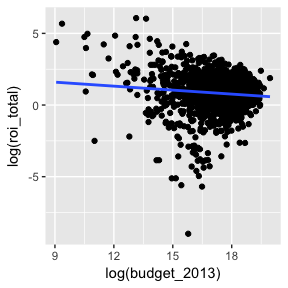roi_vs_budget <- lm(log(roi_total) ~ log(budget_2013),
data = bechdel90_13)
tidy(roi_vs_budget)
term estimate std.error statistic p.value
(Intercept) 2.4300342 0.3898688 6.232953 0.0000000
log(budget_2013) -0.0926098 0.0225334 -4.109890 0.0000416

Note the negative coefficient here on log(budget_2013) and its corresponding small $$p$$-value.

### Bechdel dummy is not a significant predictor of log(roi_total) assuming log(budget_2013) is in the model

Note that the regression lines nearly completely overlap.

ggplot(data = bechdel90_13,
mapping = aes(x = log(budget_2013), y = log(roi_total),
color = binary)) +
geom_point() +
geom_smooth(method = "lm", se = FALSE)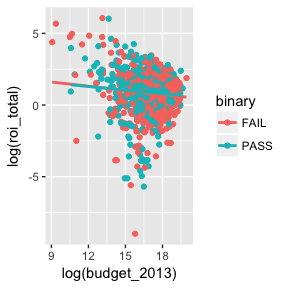roi_vs_budget_binary <- lm(log(roi_total) ~ log(budget_2013) + factor(binary),
data = bechdel90_13)
tidy(roi_vs_budget_binary)
term estimate std.error statistic p.value
(Intercept) 2.3585962 0.3988812 5.9130285 0.0000000
log(budget_2013) -0.0899228 0.0227566 -3.9515046 0.0000810
factor(binary)PASS 0.0539207 0.0635194 0.8488861 0.3960713

Note the $$p$$-value on factor(binary)PASS here that is around 0.40.

## Dollars Earned for Every Dollar Spent graphic

Calculating the values and creating a tidy data frame

passes_bechtel_rom <- bechdel90_13 %>%
filter(generous == TRUE) %>%
summarize(median_roi = median(roi_dom, na.rm = TRUE))
median_groups_dom <- bechdel90_13 %>%
filter(clean_test %in% c("men", "notalk", "nowomen")) %>%
group_by(clean_test) %>%
summarize(median_roi = median(roi_dom, na.rm = TRUE))
pass_bech_rom <- data_frame(clean_test = "pass",
median_roi = passes_bechtel_rom$median_roi) med_groups_dom_full <- bind_rows(pass_bech_rom, median_groups_dom) %>% mutate(group = "U.S. and Canada") ## Warning in bind_rows_(x, .id): binding character and factor vector, ## coercing into character vector passes_bechtel_int <- bechdel90_13 %>% filter(generous == TRUE) %>% summarize(median_roi = median(roi_int, na.rm = TRUE)) median_groups_int <- bechdel90_13 %>% filter(clean_test %in% c("men", "notalk", "nowomen")) %>% group_by(clean_test) %>% summarize(median_roi = median(roi_int, na.rm = TRUE)) pass_bech_int <- data_frame(clean_test = "pass", median_roi = passes_bechtel_int$median_roi)
med_groups_int_full <- bind_rows(pass_bech_int, median_groups_int) %>%
mutate(group = "International")
## Warning in bind_rows_(x, .id): binding character and factor vector,
## coercing into character vector
med_groups <- bind_rows(med_groups_dom_full, med_groups_int_full) %>%
mutate(clean_test = str_replace_all(clean_test,
"pass",
"Passes Bechdel Test"),
clean_test = str_replace_all(clean_test, "men",
clean_test = str_replace_all(clean_test, "notalk",
"Women don't talk to each other"),
clean_test = str_replace_all(clean_test, "nowoWomen only talk about men",
"Fewer than two women"))
med_groups %<>% mutate(clean_test = factor(clean_test,
levels = c("Fewer than two women",
"Women don't talk to each other",
"Passes Bechdel Test"))) %>%
mutate(group = factor(group, levels = c("U.S. and Canada", "International"))) %>%
mutate(median_roi_dol = dollar(median_roi))

Using only a few functions to plot

ggplot(data = med_groups, mapping = aes(x = clean_test, y = median_roi,
fill = group)) +
geom_bar(stat = "identity") +
facet_wrap(~ group) +
coord_flip() +
labs(title = "Dollars Earned for Every Dollar Spent", subtitle = "2013 dollars") +
scale_fill_fivethirtyeight() +
theme_fivethirtyeight()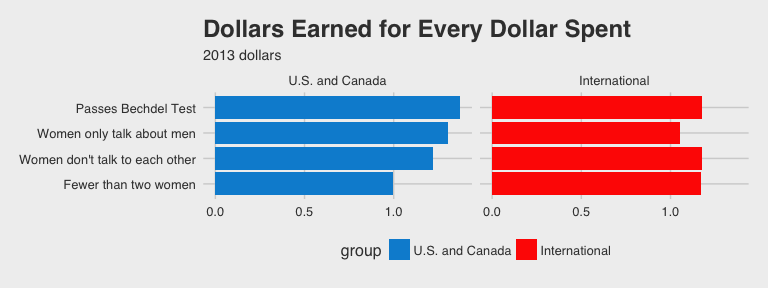Attempt to fully reproduce Dollars Earned for Every Dollar Spent plot using ggplot

ggplot(data = med_groups, mapping = aes(x = clean_test, y = median_roi,
fill = group)) +
geom_bar(stat = "identity") +
geom_text(aes(label = median_roi_dol), hjust = -0.1) +
scale_y_continuous(expand = c(.25, 0)) +
coord_flip() +
facet_wrap(~ group) +
scale_fill_manual(values = c("royalblue", "goldenrod")) +
labs(title = "Dollars Earned for Every Dollar Spent", subtitle = "2013 dollars") +
theme_fivethirtyeight() +
theme(plot.title = element_text(hjust = -1.6),
plot.subtitle = element_text(hjust = -0.4),
strip.text.x = element_text(face = "bold", size = 16),
panel.grid.major = element_blank(),
panel.grid.minor = element_blank(),
axis.title.x = element_blank(),
axis.text.x = element_blank(),
axis.ticks.x = element_blank()) +
guides(fill = FALSE)